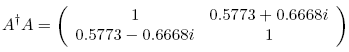occasional meanderings in physics' brave new world

Friday, November 14, 2008

M Theory Lesson 237

The tribimaximal mixing matrix $T$, which is easily expressed in circulant form, is Fourier transformed to a block matrixwhich has exact entries

$\frac{2 \sqrt{2} - 1}{2 \sqrt{6}} \pm \frac{\sqrt{3}}{2 \sqrt{6}} i$
$\frac{\sqrt{3} + \sqrt{6}}{2 \sqrt{6}} \pm \frac{1 - \sqrt{2}}{2 \sqrt{6}} i$
$\frac{1 + \sqrt{2}}{\sqrt{6}} + \frac{1 - \sqrt{2}}{\sqrt{6}} i$

Numerically, this transform takes the formObserve that the norm of both the $GL(1)$ component and the $GL(2)$ component is 1, as in the case of the CKM transform. This reduces the parameterisation to four real parameters. The two dimensional component $A$ does not belong to $SU(2)$, but $A^{\dagger} A$ is a braided circulant, namelywhere the imaginary part should be exactly $2/3$, but my rounding was lazy. The real part is exactly $\sqrt{3}^{-1}$, and the determinant of $A^{\dagger} A$ is $2/9$ (a number that M theorists will recognise).CarlBrannen said...

Marni, I'm going to explore these things numerically as soon as I get enough stuff listed on eBay that I am assured I have my December rent.

November 15, 2008 7:50 AMKea said...

OK, Carl, sounds like a good plan. I already have my December rent sorted. Now I'm saving so I can go to the dentist.

November 15, 2008 1:16 PMCarlBrannen said...

Okay, the first night didn't get anything. The problem is that there are too much degrees of freedom.

So I'm going to try another night of it, tonight (i.e. over the next 8 hours).

November 16, 2008 2:35 PM